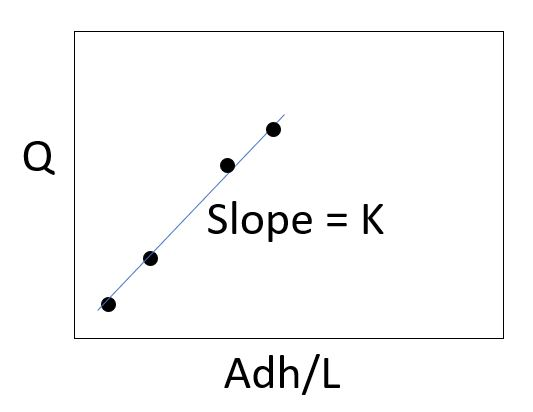# Darcy Column Test for Permeability

In this simulation you will perform a constant head permeability test on fine gravel. This is a diagram of the system used in the videoWater is pumped from a reservoir into the top of the soil column. The column has a radius (R) of 2.54 cm amd length (L) of 24.5 cm. An overflow at the top of the column will keep the water at a constant head (h1 = 24.5 cm). A manometer that measures the head at the bottom of the soil column is attached to the system outflow. Owing to friction, the head at the bottom of the column will be less than that at the top (i.e., there is an energy drop) and will be proportional to the flow rate as described by Darcy’s Law. Q = KAdh/L where Q = flow rate (cc/s) A is the cross sectional area (pi*R2 ) (cm2) dh = head drop = h1-h2 ( h1 = 24/.5) (in cm) L = column length of soil (24.5 cm) K = hydraulic Conductivity (cm/s)To determine K the following procedure is used. The valve at the bottom of the column will be used to set a flow rate. To determine the flow rate, you will be recording the amount of time it takes to fill a graduated cylinder to some volume and calculating the Q = Volume/time. You will also record the h2 value in the manometer. These values will be put in a data collection and analysis spreadsheet to make the following plot. A best fit line will be fit to the data. The slope of the line equals the hydraulic conductivity. To run the test open the data collection and analysis spreadsheet. Have your timer ready. Then start the video. After enter your results below. Data Collection and Analysis Spreadsheet

### Darcy Column test results

• MM slash DD slash YYYY
• Convert cm/s to feet/day. There are 30.48 cm per foot and 86400 seconds in a day.
• Accepted file types: pdf, Max. file size: 100 MB.
Be sure to save the spreadsheet as a pdf with your last name in the file name. e.g. Robbins-DataCollectionandAnalysis.pdf
• This field is for validation purposes and should be left unchanged.